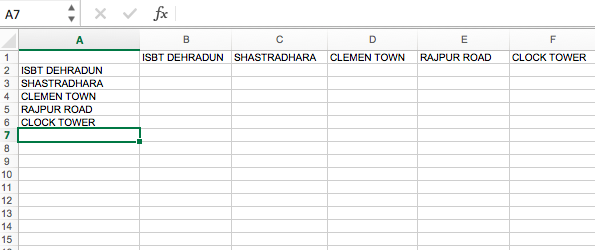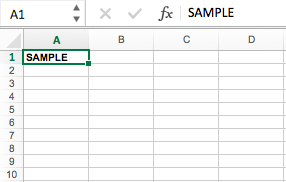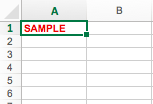# Writing to an excel sheet using Python

## Writing to an excel sheet using Python

Using `xlwt` module, one can perform multiple operations on spreadsheet. For example, writing or modifying the data can be done in Python. Also, the user might have to go through various sheets and retrieve data based on some criteria or modify some rows and columns and do a lot of work.

Let’s see how to create and write to an excel-sheet using Python.

Code #1 :

 `# Writing to an excel ` `# sheet using Python` `import` `xlwt` `from` `xlwt ``import` `Workbook` ` ` `# Workbook is created` `wb ``=` `Workbook()` ` ` `# add_sheet is used to create sheet.` `sheet1 ``=` `wb.add_sheet(``'Sheet 1'``)` ` ` `sheet1.write(``1``, ``0``, ``'ISBT DEHRADUN'``)` `sheet1.write(``2``, ``0``, ``'SHASTRADHARA'``)` `sheet1.write(``3``, ``0``, ``'CLEMEN TOWN'``)` `sheet1.write(``4``, ``0``, ``'RAJPUR ROAD'``)` `sheet1.write(``5``, ``0``, ``'CLOCK TOWER'``)` `sheet1.write(``0``, ``1``, ``'ISBT DEHRADUN'``)` `sheet1.write(``0``, ``2``, ``'SHASTRADHARA'``)` `sheet1.write(``0``, ``3``, ``'CLEMEN TOWN'``)` `sheet1.write(``0``, ``4``, ``'RAJPUR ROAD'``)` `sheet1.write(``0``, ``5``, ``'CLOCK TOWER'``)` ` ` `wb.save(``'xlwt example.xls'``)`

Output :Code #2 : Adding style sheet in excel

 `# importing xlwt module` `import` `xlwt` ` ` `workbook ``=` `xlwt.Workbook() ` ` ` `sheet ``=` `workbook.add_sheet(``"Sheet Name"``)` ` ` `# Specifying style` `style ``=` `xlwt.easyxf(``'font: bold 1'``)` ` ` `# Specifying column` `sheet.write(``0``, ``0``, ``'SAMPLE'``, style)` `workbook.save(``"sample.xls"``)`

Output :Code #3 : Adding multiple styles to a cell

 `# importing xlwt module` `import` `xlwt` ` ` `workbook ``=` `xlwt.Workbook() ` ` ` `sheet ``=` `workbook.add_sheet(``"Sheet Name"``)` ` ` `# Applying multiple styles` `style ``=` `xlwt.easyxf(``'font: bold 1, color red;'``)` ` ` `# Writing on specified sheet` `sheet.write(``0``, ``0``, ``'SAMPLE'``, style)` ` ` `workbook.save(``"sample.xls"``)`

Output :Last Updated on October 28, 2021 by admin

## Python – Add image on a Tkinter buttonPython – Add image on a Tkinter button

Python | Add image on a Tkinter button Tkinter is a Python module which is used

## Python – Perform append at beginning of listPython – Perform append at beginning of list

Python | Perform append at beginning of list   The usual append operation of Python

## Remove multiple elements from a list in PythonRemove multiple elements from a list in Python

Remove multiple elements from a list in Python   Given a list of numbers, write

## Data Wrangling in PythonData Wrangling in Python

Data Wrangling in Python Data Wrangling is the process of gathering, collecting, and transforming Raw

## Packing and Unpacking Arguments in PythonPacking and Unpacking Arguments in Python

Packing and Unpacking Arguments in Python We use two operators * (for tuples) and **

## Load CSV data into List and Dictionary using PythonLoad CSV data into List and Dictionary using Python

Load CSV data into List and Dictionary using Python CSV (Comma Separated Values) is a simple

## Python – Create a directoryPython – Create a directory

Create a directory in Python The OS module in Python provides functions for interacting with

## Python – Difference between two listsPython – Difference between two lists

Python – Difference between two lists   There are various ways in which the difference